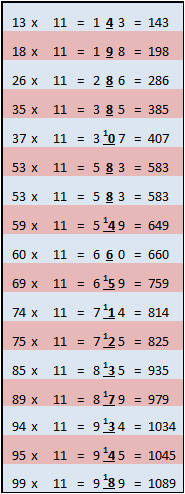Quick Multiplication by 11

This is an easy way to multiply numbers by 11

Case 1 (Simple 2 digit numbers)
Let us use 34 for example
1)  Split the two digits in your head

3X4
The answer will look like that

2) To figure what the X is
Add the 3 and the 4 together (3+4=7)
Replace the X by this sum(7)
374

Case 2 (2 digit numbers with a carry)
In this case the sum of the two numbers is greater than 9

Let us use 76 for example

1)  Split the two digits in your head
7X6
The answer will look like that
2)   To figure what the X is
Add the 7 and the 6 together (7+6=13)
Replace the X by this sum (13)
7136

3) Clearly this answer is not correct, as it has to be a 3 digit number.
Take the extra 1 that has occurred from the sum being greater than 9 and add it to the 100s number (7)
7136   ->   7136   ->  836